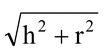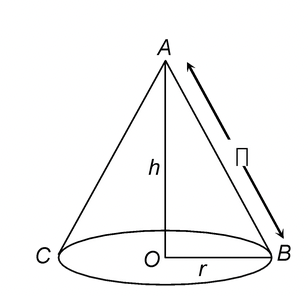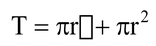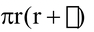# SURFACE AREA OF A RIGHT CIRCULAR CONE

## CURVED SURFACE AREA OF A CONE:

C = π rh

C = curved surface area

r = radius of base of cone

h = slant height

c =### TOTAL SURFACE AREA OF A CONE:=Here, T = total surface area

r = radius of base of cone

h = slant height of cone